# Sturm-Liouville theory

In mathematics and its applications, a classical Sturm–Liouville equation is a real second-order linear differential equation of the form: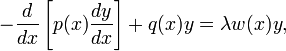$-\frac{d}{dx}\left[p(x)\frac{dy}{ dx}\right]+q(x)y=\lambda w(x)y,$

(1)

where y is a function of the free variable x. Here the functions p(x) > 0 has a continuous derivative, q(x), and w(x) > 0 are specified at the outset, and in the simplest of cases are continuous on the finite closed interval [a,b]. In addition, the function y is typically required to satisfy some boundary conditions at a and b. The function w(x), which is sometimes called r(x), is called the "weight" or "density" function. The equation is named after Jacques Charles François Sturm (1803–1855) and Joseph Liouville (1809–1882).

The value of λ is not specified in the equation; finding the values of λ for which there exists a non-trivial solution of (1) satisfying the boundary conditions is part of the problem called the Sturm–Liouville problem (S–L).

Such values of λ when they exist are called the eigenvalues of the boundary value problem defined by (1) and the prescribed set of boundary conditions. The corresponding solutions (for such a λ) are the eigenfunctions of this problem. Under normal assumptions on the coefficient functions p(x), q(x), and w(x) above, they induce a Hermitian differential operator in some function space defined by boundary conditions. The resulting theory of the existence and asymptotic behavior of the eigenvalues, the corresponding qualitative theory of the eigenfunctions and their completeness in a suitable function space became known as Sturm–Liouville theory. This theory is important in applied mathematics, where S–L problems occur very commonly, particularly when dealing with linear partial differential equations that are separable.

##  Sturm–Liouville theory

Under the assumptions that the S–L problem is regular, that is, p(x)−1 > 0, q(x), and w(x) > 0 are real-valued integrable functions over the finite interval [ab], with separated boundary conditions of the form:$y(a)\cos \alpha - p(a)y^\prime (a)\sin \alpha = 0,$

(2)$y(b)\cos \beta - p(b)y^\prime (b)\sin \beta = 0,$

(3)

where$\alpha, \beta \in [0, \pi),$ the main tenet of Sturm–Liouville theory states that:

• The eigenvalues λ1, λ2, λ3, ... of the regular Sturm–Liouville problem (1) - (2) - (3) are real and can be ordered such that: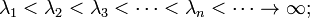$\lambda_1 < \lambda_2 < \lambda_3 < \cdots < \lambda_n < \cdots \to \infty; \,$
• Corresponding to each eigenvalue λn is a unique (up to a normalization constant) eigenfunction yn(x) which has exactly n − 1 zeros in (ab). The eigenfunction yn(x) is called the n-th fundamental solution satisfying the regular Sturm–Liouville problem (1) - (2) - (3).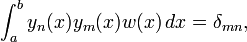$\int_a^b y_n(x)y_m(x)w(x)\,dx = \delta_{mn},$
in the Hilbert space L2([ab],w(xdx). Here δmn is a Kronecker delta.

Since by assumption the eigenfunctions are normalized, the result is established by a proof of their orthogonality.

Note that, unless p(x) is continuously differentiable and q(x), w(x) are continuous, the equation has to be understood in a weak sense.

##  Sturm–Liouville form

The differential equation (1) is said to be in Sturm–Liouville form or self-adjoint form. All second-order linear ordinary differential equations can be recast in the form on the left-hand side of (1) by multiplying both sides of the equation by an appropriate integrating factor (although the same is not true of second-order partial differential equations, or if y is a vector.)

###  Examples

The Bessel equation:$x^2y''+xy'+(\lambda^2x^2-\nu^2)y=0\,$

can be written in Sturm–Liouville form as:$(xy')'+(\lambda^2 x-\nu^2/x)y=0.\,$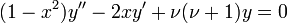$(1-x^2)y''-2xy'+\nu(\nu+1)y=0\;\!$

can easily be put into Sturm–Liouville form, since D(1 − x2) = −2x, so, the Legendre equation is equivalent to: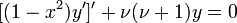$[(1-x^2)y']'+\nu(\nu+1)y=0\;\!$

It takes more work to put the following differential equation into Sturm–Liouville form: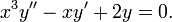$x^3y''-xy'+2y=0.\,$

Divide throughout by x3: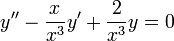$y''-{x\over x^3}y'+{2\over x^3}y=0$

Multiplying throughout by an integrating factor of: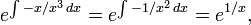$e^{\int -{x / x^3}\,dx}=e^{\int -{1 / x^2}\, dx}=e^{1 / x},$

gives: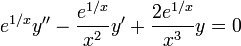$e^{1 / x}y''-{e^{1 / x} \over x^2} y'+ {2 e^{1 / x} \over x^3} y = 0$

which can be easily put into Sturm–Liouville form since:$D e^{1 / x} = -{e^{1 / x} \over x^2}$

so the differential equation is equivalent to: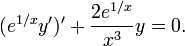$(e^{1 / x}y')'+{2 e^{1 / x} \over x^3} y =0.$

In general, given a differential equation: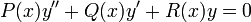$P(x)y''+Q(x)y'+R(x)y=0\,$

dividing by P(x), multiplying through by the integrating factor:$e^{\int {Q(x) / P(x)}\,dx},$

and then collecting gives the Sturm–Liouville form.

##  Sturm–Liouville equations as self-adjoint differential operators

Let us rewrite equation (1) as$\Lambda\,y(x) = \lambda\,w(x)\,y(x)$

(1a)

with$\Lambda \equiv \left(-{d\over dx}\left[p(x){d\over dx}\right]+q(x) \right).$

The function w(x) is positive-definite and hence equation (1a) has the form of a generalized operator eigenvalue equation. It can be transformed to a regular eigenvalue equation by substitution of$u(x) = w(x)^{1/2} y (x)\quad\hbox{and}\quad L = w(x)^{-1/2}\Lambda w(x)^{-1/2}.$

Equation (1a) becomes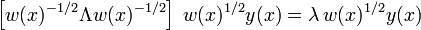$\left[w(x)^{-1/2} \Lambda w(x)^{-1/2}\right] \; w(x)^{1/2} y(x) = \lambda\, w(x)^{1/2}y(x)$

or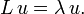$L\, u = \lambda\,u.$

(1b)

The map L can be viewed as a linear operator mapping a function u to another function Lu. We may study this linear operator in the context of functional analysis. Equation (1b) is precisely the eigenvalue problem of L; that is, we are trying to find the eigenvalues λ1, λ2, λ3, ... and the corresponding eigenvectors u1, u2, u3, ... of the L operator. The proper setting for this problem is the Hilbert space L2([ab],w(xdx) with scalar product:$\langle u_i, u_j\rangle = \int_{a}^{b} \overline{u_i(x)} u_j(x) \,dx = \int_{a}^{b} \overline{y_i(x)} y_j(x) \, w(x)\, dx, \quad u_{k}(x) \equiv w(x)^{1/2} y_k(x).$

The functions y solve the generalized eigenvalue problem (1a) and the functions u the ordinary eigenvalue problem (1b).

In this space L is defined on sufficiently smooth functions which satisfy the above boundary conditions. Moreover, L is a self-adjoint operator. This can be seen formally by using integration by parts twice, where the boundary terms vanish by virtue of the boundary conditions. The functions w(x), p(x), and q(x) are real. From the vanishing of the boundary terms follows (d/dx) = − d/dx, hence,$L^* = (w^{-1/2} \Lambda w^{-1/2})^* = w^{-1/2} \Lambda^* w^{-1/2} \quad\hbox{and}\quad \Lambda^* = \left(-{d\over dx}\left[p(x){d\over dx}\right]+q(x) \right)^* = \left(-{d\over dx}\left[p(x){d\over dx}\right]+q(x) \right).$

Both L and Λ are self-adjoint. It then follows that the eigenvalues λ shared by L and Λ are real and that eigenfunctions of L corresponding to different eigenvalues are orthogonal. If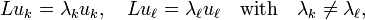$Lu_k = \lambda_k u_k, \quad Lu_\ell = \lambda_\ell u_\ell \quad\hbox{with}\quad \lambda_k \ne \lambda_\ell,$

then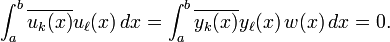$\int_{a}^{b} \overline{u_k(x)} u_\ell(x) \,dx = \int_{a}^{b} \overline{y_k(x)} y_\ell(x) \, w(x)\, dx = 0.$

However, the operator L is unbounded and hence existence of an orthonormal basis of eigenfunctions is not evident. To overcome this problem one looks at the resolvent: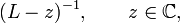$(L - z)^{-1}, \qquad z \in\mathbb{C},$

where z is chosen to be some complex number which is not an eigenvalue. Then, computing the resolvent amounts to solving the inhomogeneous equation, which can be done using the variation of parameters formula. This shows that the resolvent is an integral operator with a continuous symmetric kernel (the Green's function of the problem). As a consequence of the Arzelà–Ascoli theorem this integral operator is compact and existence of a sequence of eigenvalues αn which converge to 0 and eigenfunctions which form an orthonormal basis follows from the spectral theorem for compact operators. Finally, note that (Lz) − 1u = αu is equivalent to Lu = (z + α − 1)u.

If the interval is unbounded, or if the coefficients have singularities at the boundary points, one calls L singular. In this case the spectrum no longer consists of eigenvalues alone and can contain a continuous component. There is still an associated eigenfunction expansion (similar to Fourier series versus Fourier transform). This is important in quantum mechanics, since the one-dimensional Schrödinger equation is a special case of a S–L equation.

##  Example

We wish to find a function u(x) which solves the following Sturm–Liouville problem:$L u = \frac{d^2u}{dx^2} = \lambda u$

(4)

where the unknowns are λ and u(x). As above, we must add boundary conditions, we take for example:$u(0) = u(\pi) = 0 \,$

Observe that if k is any integer, then the function:$u(x) = \sin kx \,$

is a solution with eigenvalue λ = −k2. We know that the solutions of a S–L problem form an orthogonal basis, and we know from the theory of Fourier series that this set of sinusoidal functions is an orthogonal basis. Since orthogonal bases are always maximal (by definition) we conclude that the S–L problem in this case has no other eigenvectors.

Given the preceding, let us now solve the inhomogeneous problem:$L u =x, \qquad x\in(0,\pi)$

with the same boundary conditions. In this case, we must write f(x) = x in a Fourier series. The reader may check, either by integrating ∫exp(ikx)x dx or by consulting a table of Fourier transforms, that we thus obtain:$L u =\sum_{k=1}^{\infty}-2\frac{(-1)^k}{k}\sin kx.$

This particular Fourier series is troublesome because of its poor convergence properties. It is not clear a priori whether the series converges pointwise. Because of Fourier analysis, since the Fourier coefficients are "square-summable", the Fourier series converges in L2 which is all we need for this particular theory to function. We mention for the interested reader that in this case we may rely on a result which says that Fourier's series converges at every point of differentiability, and at jump points (the function x, considered as a periodic function, has a jump at π) converges to the average of the left and right limits (see convergence of Fourier series).

Therefore, by using formula (4), we obtain the solution: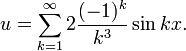$u=\sum_{k=1}^{\infty}2\frac{(-1)^k}{k^3}\sin kx.$

In this case, we could have found the answer using antidifferentiation. This technique yields u = (x3 − π2x)/6, whose Fourier series agrees with the solution we found. The antidifferentiation technique is not generally useful when the differential equation has many variables.

##  Application to normal modes

Suppose we are interested in the modes of vibration of a thin membrane, held in a rectangular frame, 0 < x < L1, 0 < y < L2. We know the equation of motion for the vertical membrane's displacement, W(x, y, t) is given by the wave equation: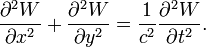$\frac{\partial^2W}{\partial x^2}+\frac{\partial^2W}{\partial y^2} = \frac{1}{c^2}\frac{\partial^2W}{\partial t^2}.$

The equation is separable (substituting W = X(x) × Y(y) × T(t)), and the normal mode solutions that have harmonic time dependence and satisfy the boundary conditions W = 0 at x = 0, L1 and y = 0, L2 are given by: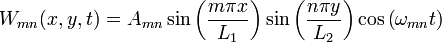$W_{mn}(x,y,t) = A_{mn}\sin\left(\frac{m\pi x}{L_1}\right)\sin\left(\frac{n\pi y}{L_2}\right)\cos\left(\omega_{mn}t\right)$

where m and n are non-zero integers, Amn is an arbitrary constant and: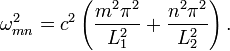$\omega^2_{mn} = c^2 \left(\frac{m^2\pi^2}{L_1^2}+\frac{n^2\pi^2}{L_2^2}\right).$

Since the eigenfunctions Wmn form a basis, an arbitrary initial displacement can be decomposed into a sum of these modes, which each vibrate at their individual frequencies ωmn. Infinite sums are also valid, as long as they converge.Some content on this page may previously have appeared on Citizendium.